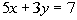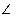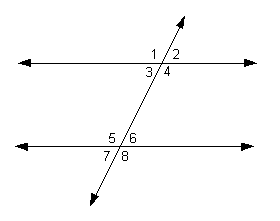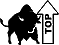TitleBeginning Algebra
Tutorial 36: Practice Test on Tutorials 32 - 35Learning Objectives

 After completing this tutorial, you should be able to: Take a test on topics covered in tutorials 32 - 35 in this website.

 Special Notes about Tutorial 36: I can not guarantee you will pass your test after going though any of the tutorials in this website or this practice test. However, it will definitely help you to better understand the topics covered better. Disclaimer: WTAMU and Kim Seward are not responsible for how a student does on any test or any class for any reason including not being able to access the website due to any technology problems.  There are no videos on this page.Introduction

It is important to note that, chances are, I'm not your math instructor. You need to check with your math teacher as to things like when your next math test is and what it covers.  It may cover more material on the test than what is in this practice test.  Just note that there are other practice tests at this website. So, after finding out what is on your test (if you have one) do the practice test(s) problems that go with the test you are preparing for.  If you are not in a class or are not having a test soon, this practice test is still good practice to go through and check to make sure you are understanding this material before moving on - kind of like a spot check. The material on this practice test goes with Tutorial 32: Formulas, Tutorial 33: Basic Geometry, Tutorial 34: Central Tendencies, and  Tutorial 35: Reasoning Skills.

Also note that your teacher may word the problems on their test a little differently, may have some different kinds of problems, or may have a different number of problems than what is in this practice test.  Again, since I'm probably not your math instructor, I don't know exactly how your teacher will set up your math test.  Just note that these problems will help you build an understanding of the concepts presented and the terms used in math problems.  If you have an understanding of the problems instead of just memorizing them, then you should do fine on these concepts, no matter how the test is set up.

 Steps to Studying for a Math Test
1. Work through problems.  If you are in a class, you should have done this on completion of any homework you have done.  For anyone,  you can accomplish this by doing the practice problems found in each tutorial.

2. Check work on problems.  The practice problems in each tutorial have links to the answers to them so you can instantly check how you are doing.  Also, in most math books, the odd answers are found in the back of the book.

3. Review concepts.  Whether you got the problems right or wrong, make sure you review over them.  If you did get a problem wrong, make sure you either review that concept in it's respective tutorial or ask your math teacher about it.  If you don't ask about a problem before a test,  you are going to kick yourself when it comes up on the test.

4. Work through problems as if you were taking the test - no notes, book, webpages, etc.  This practice test is a perfect way to do that. After taking this practice test, check your answers by clicking on the link to the answer key found at the bottom of the practice test (before the 'need extra help on these topics' section)

 During the Test

It is to your benefit to show as much of the work as possible on the problems that have several steps involved.

Make sure that you read the directions carefully,  you wouldn't believe how many points get taken off  math tests for people not following directions.

Pace yourself.  You do not have to be the first one done to do well on the test.  Do not panic if there is still time left to take the test and others are turing it in.  Sometimes that means they do not know the material and left some of the answers blank.  Do not worry about anyone else but yourself.

Don't rush through a problem.  Another thing that math teachers take points off for are careless mistakes made by people that rush through a problem.  When those students get their tests back, they bonk themselves on the head at some of the things that got counted wrong,  things that they knew how to do.

Remember to breathe!!!!  I know some of you are scared to death at the thought of having to take a math test of any kind.  For you guys, try to relax and don't forget to breathe.  (Even if you aren't scared to take a math test,  it is probably a good idea to remember to breathe,  I wouldn't want you to pass out during the test). If it feels like your brain has left the building during your test, just close your eyes and breathe in and out and in and out and your brain will return.

Good luck on your test.  If you are taking a math test soon, don't panic, you are going to do great!!!Practice Test

 Problems 1a - 1b: Solve each equation for the specified variable.

 1a.for y 1b.  I = PRT;   for P

 Problems 2a - 2b: Solve the following word problems.

 2a.  A ramp 5 feet long is leaning against a raised platform which is 4 feet above the ground.  What is the distance from the ramp's contact point with the ground and the base of the platform?

 2b.  A farmer has three cylindrical shaped containers to hold feed in.  Each container has the same radius of 3 feet and height of 4 feet.  What is the total volume of the three containers? Hint: the volume formula for a cylinder isProblem 3a: Answer the question on complimentary angles.

 3a.   What is the complementary angle to 47 degrees?

 Problem 4a: Answer the question on supplementary angles.

 4a.  What is the supplementary angle to 47 degrees?

 Problem 5a: Answer the question on congruent figures.

 5a.  Figure ABCD is congruent to figure EFGH. IfB = 70,C = 55, andD = 25, what is the measure ofH?  If AD = 25, EF = 35, and BC = 12, what is the length of EH?

 Problem 6a: Answer the question on similar figures.

 6a.  Figure ABCD is similar to figure EFGH. IfB = 60,C = 50, andD = 45, what is the measure ofG?  If AD = 25, EH = 50, and BC = 10, what is the length of FG?

 Problems 7a - 7d: Use the following figure to answer the questions.7a.   Find2 if7 = 75 degrees. 7b.  Find3 if6 = 75 degrees. 7c.  Find1 if5 = 105 degrees. 7d.  Find4 if6 = 75 degrees.

 Problem 8a: Find the mean, median, and mode.

8a.  The number of points a receiver has made during the last 5 games is given in the following table:

 Game game 1 game 2 game 3 game 4 game 5 Points 12 6 12 12 18

Find the mean, median, and mode of the points he scored in a game.

 Problem 9a: Find the test score.

 9a.  A student received scores of 62, 75, and 77 on three quizzes.  If tests count twice as much as quizzes, what is the lowest score that the student can get on the next test to achieve a mean of at least 70?

 Problems 10a - 10b: Write the next three numbers in the sequence.

 10a.   1, 3, 7, 13, 21, ... 10b.  1, 5, 25, 125, ...

 Problem 11a: Sara, Trudy, Jill and Karen all work for the same company.  One is a secretary, one is a manager, one is a computer programmer and one is a engineer.  Use the statements below to answer the question that follows.

 11a. A.   Sara and Jill eat lunch with the manager. B.   Trudy and Karen carpool with the secretary. C.   Sara likes to work out with the engineer and the secretary. Who is the secretary?Need Extra Help on these Topics?

The following are web pages that can assist you in the topics that were covered on this page:

 Tutorial 32: Formulas This tutorial will help you with problems 1a - 1b and 2a - 2b on this practice test. Tutorial 33: Basic Geometry This tutorial will help you with problems 3a, 4a, 5a, 6a and 7a - 7d on this practice test. Tutorial 34: Central Tendencies This tutorial will help you with problems 8a and 9a on this practice test. Tutorial 35: Reasoning Skills This tutorial will help you with problems 10a - 10b and 11a on this practice test.

Go to Get Help Outside the Classroom found in Tutorial 1: How to Succeed in a Math Class for some more suggestions.Last revised on August 7, 2011 by Kim Seward.